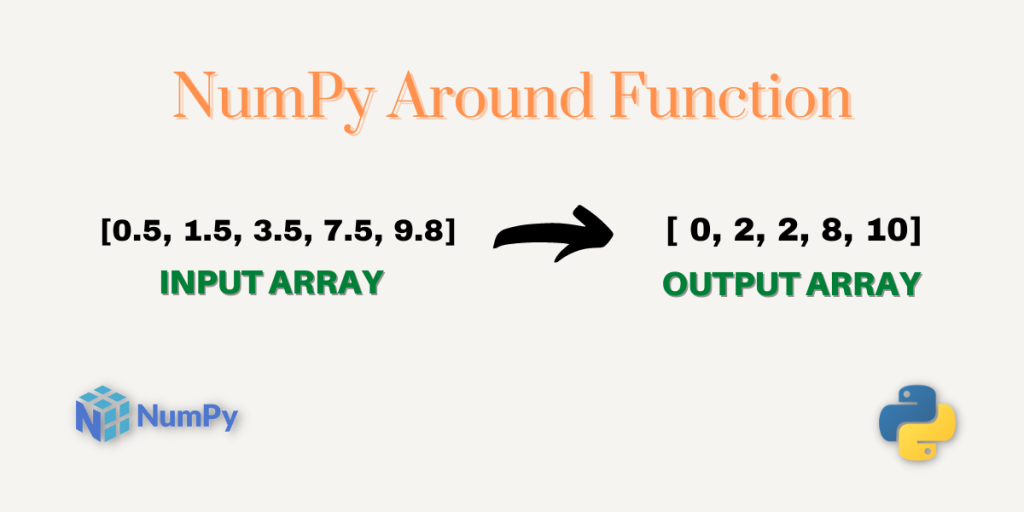# NumPy around – A Complete GuideWelcome to another tutorial on NumPy Mathematical Functions. In this tutorial, we will learn how to use the `NumPy around` function in detail, we will also practice a variety of examples to make our understanding clear.

We all must have solved different types of problems in Mathematics or Physics in which the problem stated that round the final answer to 2 decimal places. Let’s say we have to round 1.7, we would think that 1.7 is nearest to 2 so after rounding off 1.7 the value will be 2.

We can do this using programming as well which we will be learning through this tutorial. So, without any further due let’s start.

NumPy exp – A Complete Guide

## What is NumPy around?

NumPy around is one of the mathematical functions of the NumPy library which rounds the number passed as input to the function.

Let’s look at the syntax of the NumPy around function.

## Syntax of NumPy around

```numpy.around(a, decimals=0, out=None)
```

Let’s understand the parameters of this function.

• `a` – is the input number which needs to be rounded off. It can be a single number as well as a NumPy array of numbers.
• `decimals` – The value of the decimals is always an integer. It specifies the number of decimal places up to which we want to round the input number. It’s an optional parameter. Its default value is 0.
• `out` – It is the output of the numpy.around() function. It’s also an optional parameter.

## Working with the NumPy around

Let’s now write some code to understand this function better.

### NumPy around with a single number as input

```import numpy as np

# Rounding off some integer values
print("Around of 1 is:",np.around(1))

print("Around of 5 is:",np.around(5))

# Rounding off some decimal values
print("Around of 5.5 is:",np.around(5.5))

print("Around of 9.54 is:",np.around(9.54))

print("Around of 12.70 is:",np.around(12.70))

print("Around of 9.112 is:",np.around(9.112))

print("Around of 10.112 is:",np.around(10.112))
```

Output

```Around of 1 is: 1
Around of 5 is: 5
Around of 5.5 is: 6.0
Around of 9.54 is: 10.0
Around of 12.70 is: 13.0
Around of 9.112 is: 9.0
Around of 10.112 is: 10.0
```

In the above output, the integer numbers remain the same even after rounding off. Every other output is rounded off to a number with a precision of 1 digit after the decimal.

### NumPy around with decimals parameter

The `decimals` argument allows us to specify the number of decimal places to which we want to round the input number.

```import numpy as np

# Round to ones place
print("Around 5.145 is:",np.around(5.145 , 0))

# Round to tenths place
print("Around 5.145 is:",np.around(5.145 , 1))

# Round to hundredths place
print("Around 5.145 is:",np.around(5.145 , 2))

# Round to thousandths place
print("Around 5.145 is:",np.around(5.145 , 3))

# Returns the same number
print("Around 5.145 is:",np.around(5.145 , 10))
```

Output

```Around 5.145 is: 5.0
Around 5.145 is: 5.1
Around 5.145 is: 5.14
Around 5.145 is: 5.145
Around 5.145 is: 5.145
```

The above output is pretty much clear where the digits are rounded up to the specified value of the `decimals`.

### NumPy around with the negative value of decimals

```import numpy as np

# Round to tenths place
print("Around 455.56 is:",np.around(455.56 , -1))

# Round to hundredths place
print("Around 455.56 is:",np.around(455.56 , -2))

# Round to thousandths place
print("Around 455.56 is:",np.around(455.56 , -3))

# Round to tenths place
print("Around 455 is:",np.around(455 , -1))
```

Output

```Around 455.56 is: 460.0
Around 455.56 is: 500.0
Around 455.56 is: 0.0
Around 455 is: 460
```

If the value of the `decimals` is set to some negative value then the non-decimal digits of the input number are rounded.

Let’s understand rounding of 455.56 with decimals value set to -2. Here, -2 implies the hundredth place in the number. Now, count the hundredth place to the left of the decimal in the number, and accordingly, the number is rounded.

Note: Rounding beyond the leftmost digit of the input number will result in 0.

### NumPy around with NumPy Array

```import numpy as np

a = np.array((1 , 3 , 5 , 100 , 145 , 344 , 745))

print("\n")
print("Input Array:\n",a)
print("Result :\n",np.around(a))

print("\n")
print("Input Array:\n",a)
print("Rounded Values:\n",np.around(a , -1))

b = np.array((0.5 , 1.5 , 1.7 , 3.5 , 7.5 , 9.8))

print("\n")
print("Input Array:\n",b)
print("Rounded Values:\n",np.around(b))

c = np.array((4.567 , 13.564 , 12.334 , 1.567 , 9.485 , 4.444))

print("\n")
print("Input Array:\n",c)
print("Rounded Values:\n",np.around(c , 2))
```

Output

```Input Array:
[  1   3   5 100 145 344 745]
Result :
[  1   3   5 100 145 344 745]

Input Array:
[  1   3   5 100 145 344 745]
Rounded Values:
[  0   0   0 100 140 340 740]

Input Array:
[0.5 1.5 1.7 3.5 7.5 9.8]
Rounded Values:
[ 0.  2.  2.  4.  8. 10.]

Input Array:
[ 4.567 13.564 12.334  1.567  9.485  4.444]
Rounded Values:
[ 4.57 13.56 12.33  1.57  9.48  4.44]
```

There is one interesting thing to note that `np.around(``)` returns a new ndarray after rounding and doesn’t affect the original ndarray.

This is where the third parameter comes into play.

### Using NumPy around with the parameter out

```import numpy as np

a = np.array((1.34 , 2.56 , 3.99 , 45.45 , 100.01))

print("Original Array:\n",a)

np.around(a , out=a)

print("Array after Rounding the values:\n",a)
```

Output

```Original Array:
[  1.34   2.56   3.99  45.45 100.01]
Array after Rounding the values:
[  1.   3.   4.  45. 100.]
```

In the above program, the rounded values are stored in the original array.

That was all from my side, do execute the code discussed in the article and try using the function with different inputs as well. Do check out the official documentation of the NumPy around function.

## Reference

NumPy Documentation – NumPy around